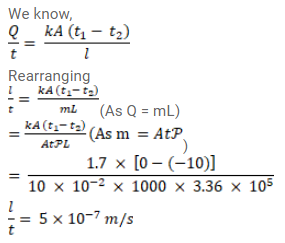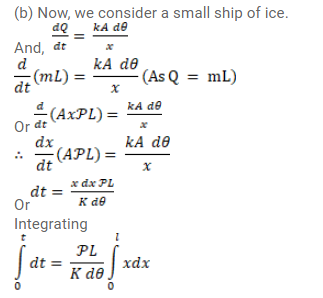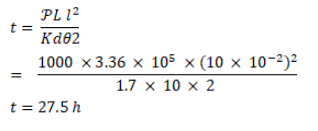# On a winter day when the atmospheric temperature drops toQuestion:

On a winter day when the atmospheric temperature drops to $-10^{\circ} \mathrm{C}$, ice forms on the surface of a lake.

(a) Calculate the rate of increase of thickness of the ice when $10 \mathrm{~cm}$ of ice is already formed.

(b) Calculate the total time taken in forming $10 \mathrm{~cm}$ of ice. Assume that the temperature of the entire water reaches $0^{\circ} \mathrm{C}$ before the ice starts forming. Density of water $=1000 \mathrm{~kg} / \mathrm{m}^{3}$ latent heat of fusion of ice $=3.36 \times 10^{5} \mathrm{~J} / \mathrm{kg}$ and thermal conductivity of ice $=1.7 \mathrm{~W} / \mathrm{m}-{ }^{\circ} \mathrm{C}$ Neglect the expansion of water on freezing.

Solution: